MathScore EduFighter is one of the best math games on the Internet today. You can start playing for free!

## Transformations - Sample Math Practice Problems

The math problems below can be generated by MathScore.com, a math practice program for schools and individual families. References to complexity and mode refer to the overall difficulty of the problems as they appear in the main program. In the main program, all problems are automatically graded and the difficulty adapts dynamically based on performance. Answers to these sample questions appear at the bottom of the page. This page does not grade your responses.

See some of our other supported math practice problems.

### Complexity=1, Mode=trans

 1.   Figure out the translation components, and then where point P' ends up: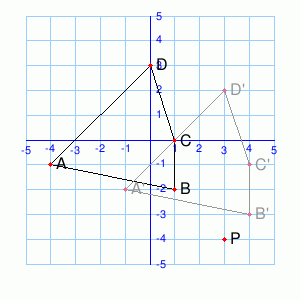Translation: ( ,) Let P = (3, -4). P' = ( ,) 2.   Figure out the translation components, and then where point P' ends up: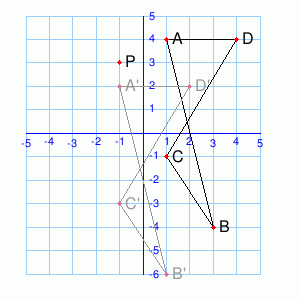Translation: ( ,) Let P = (-1, 3). P' = ( ,)

### Complexity=1, Mode=rot

 1.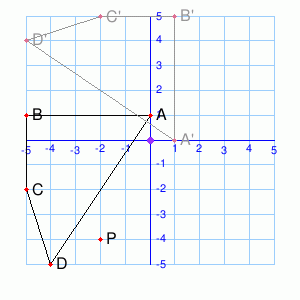The transformation ABCD → A'B'C'D' is a rotation around (0, 0) by ° Rotate P around (0, 0) by the same angle. (You may need to sketch things out on paper.) P' = ( ,) 2.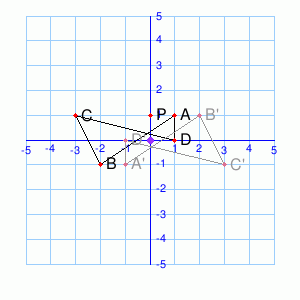The transformation ABCD → A'B'C'D' is a rotation around (0, 0) by ° Rotate P around (0, 0) by the same angle. (You may need to sketch things out on paper.) P' = ( ,)

### Complexity=1, Mode=refl

 1.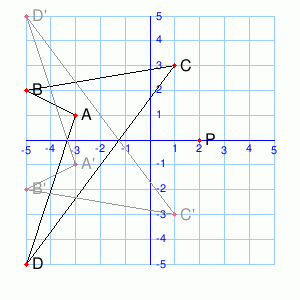Transformation ABCD → A'B'C'D' is a reflection across the        x-axis        y-axis Reflect P over the same line. (You may need to sketch things out on paper.) P' = ( ,) 2.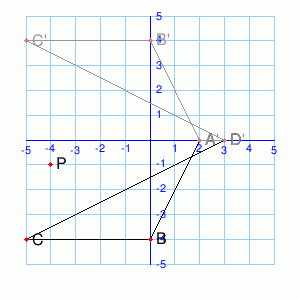Transformation ABCD → A'B'C'D' is a reflection across the        x-axis        y-axis Reflect P over the same line. (You may need to sketch things out on paper.) P' = ( ,)

### Complexity=1, Mode=mix

 1.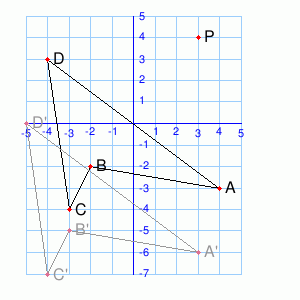What type of transform takes ABCD to A'B'C'D'?        Translation        Rotation        Reflection 2.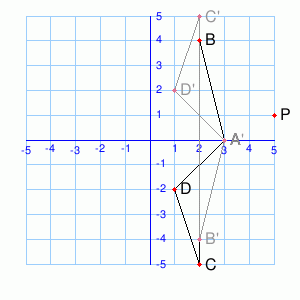What type of transform takes ABCD to A'B'C'D'?        Translation        Rotation        Reflection

### Complexity=2, Mode=rot

 1.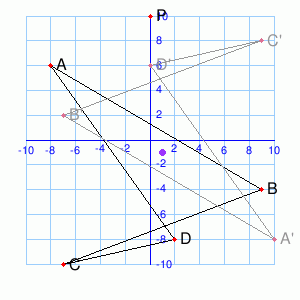The transformation ABCD → A'B'C'D' is a rotation around (1, -1) by ° Rotate P around (1, -1) by the same angle. (You may need to sketch things out on paper.) P' = ( ,) 2.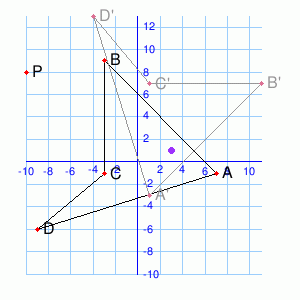The transformation ABCD → A'B'C'D' is a rotation around (3, 1) by ° Rotate P around (3, 1) by the same angle. (You may need to sketch things out on paper.) P' = ( ,)

### Complexity=2, Mode=refl

 1.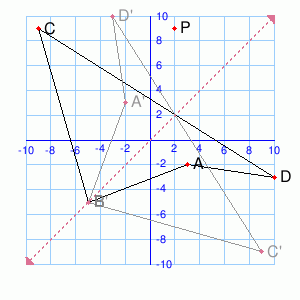Transformation ABCD → A'B'C'D' is a reflection across the line e.g. y=-3, y=x. Reflect P over the same line. (You may need to sketch things out on paper.) P' = ( ,) 2.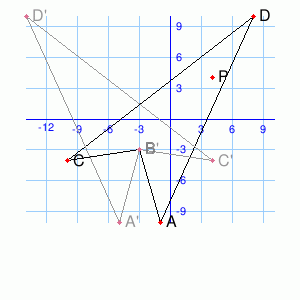Transformation ABCD → A'B'C'D' is a reflection across the line e.g. y=-3, y=x. Reflect P over the same line. (You may need to sketch things out on paper.) P' = ( ,)

### Complexity=1, Mode=trans

1Figure out the translation components, and then where point P' ends up:Translation: ( ,)
Let P = (3, -4).
P' = ( ,)
Solution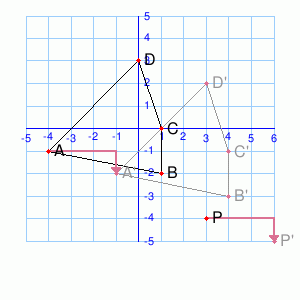To figure out the translation vector, pick a labeled point and subtract the old coordinates from the new. For example,
```Translation
A'(-1, -2)
- A (-4, -1)
-------------
(3, -1)

P' = P(3, -4)
+ (3, -1)
--------------
(6, -5)```
2Figure out the translation components, and then where point P' ends up:Translation: ( ,)
Let P = (-1, 3).
P' = ( ,)
Solution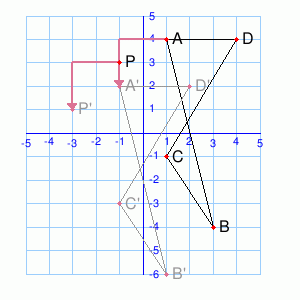To figure out the translation vector, pick a labeled point and subtract the old coordinates from the new. For example,
```Translation
A'(-1, 2)
- A (1, 4)
-------------
(-2, -2)

P' = P(-1, 3)
+ (-2, -2)
--------------
(-3, 1)```

### Complexity=1, Mode=rot

1The transformation ABCD → A'B'C'D' is a rotation around (0, 0) by °
Rotate P around (0, 0) by the same angle. (You may need to sketch things out on paper.)
P' = ( ,)
Solution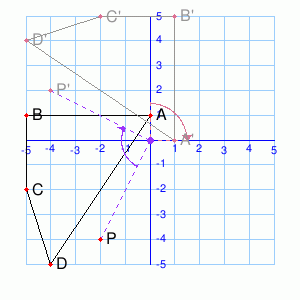Each point has been rotated around (0, 0) by -90°, which is also 270°.
A(0, 1) → A'(1, -0)
P(-2, -4) → P'(-4, 2)
Since this is a rotation by an increment of 90°, we can figure out P' graphically by forming a right triangle with its hypotenuse connecting the pivot and point P. This should make it easy to draw the rotated P'. Your before and after triangles should both have legs of length 2 and 4, since P(-2, -4) is 2 from pivot (0, 0) in the x direction and 4 from it in the y direction.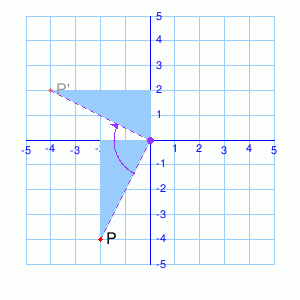2The transformation ABCD → A'B'C'D' is a rotation around (0, 0) by °
Rotate P around (0, 0) by the same angle. (You may need to sketch things out on paper.)
P' = ( ,)
Solution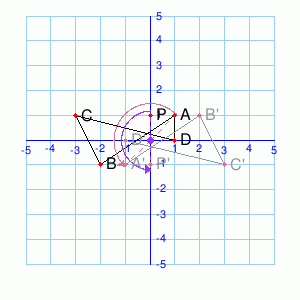Each point has been rotated around (0, 0) by 180°.
A(1, 1) → A'(-1, -1)
P(0, 1) → P'(-0, -1)
P is 1 in the y direction from the pivot. Rotating by 180 puts it at P'(-0, -1).

### Complexity=1, Mode=refl

1Transformation ABCD → A'B'C'D' is a reflection across the
x-axis        y-axis
Reflect P over the same line. (You may need to sketch things out on paper.)
P' = ( ,)
Solution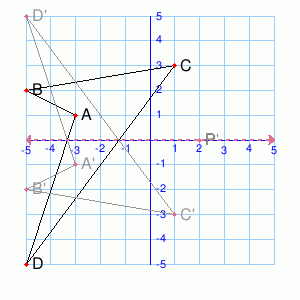The line of reflection is y = 0, the x-axis.
P is at (2, 0). Since the line of reflection is y = 0, reflecting a point comes down to switching the x and y coordinates.
2Transformation ABCD → A'B'C'D' is a reflection across the
x-axis        y-axis
Reflect P over the same line. (You may need to sketch things out on paper.)
P' = ( ,)
Solution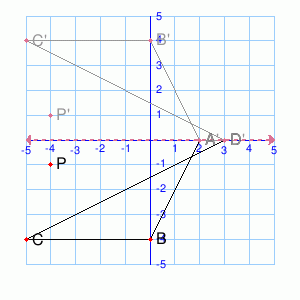The line of reflection is y = 0, the x-axis.
P is at (-4, -1). Since the line of reflection is y = 0, reflecting a point comes down to switching the x and y coordinates.

### Complexity=1, Mode=mix

1What type of transform takes ABCD to A'B'C'D'?
Translation        Rotation        Reflection
Solution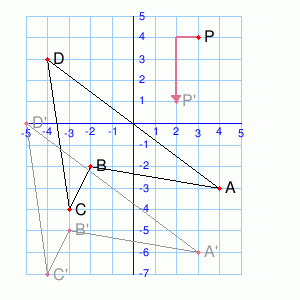Each point has been translated by (-1, -3).
2What type of transform takes ABCD to A'B'C'D'?
Translation        Rotation        Reflection
Solution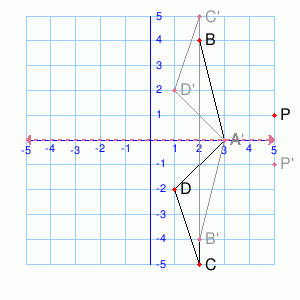Each point has been reflected across the x-axis.

### Complexity=2, Mode=rot

1The transformation ABCD → A'B'C'D' is a rotation around (1, -1) by °
Rotate P around (1, -1) by the same angle. (You may need to sketch things out on paper.)
P' = ( ,)
Solution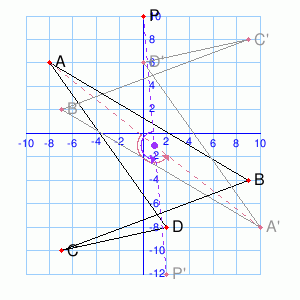Each point has been rotated around (1, -1) by 180°.
A(-8, 6) → A'(10, -8)
P(0, 10) → P'(2, -12)
Since this is a rotation by an increment of 90°, we can figure out P' graphically by forming a right triangle with its hypotenuse connecting the pivot and point P. This should make it easy to draw the rotated P'. Your before and after triangles should both have legs of length 1 and 11, since P(0, 10) is 1 from pivot (1, -1) in the x direction and 11 from it in the y direction.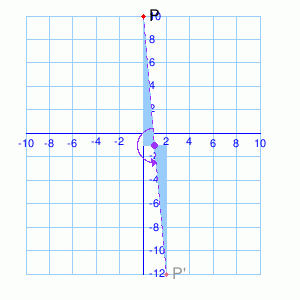2The transformation ABCD → A'B'C'D' is a rotation around (3, 1) by °
Rotate P around (3, 1) by the same angle. (You may need to sketch things out on paper.)
P' = ( ,)
Solution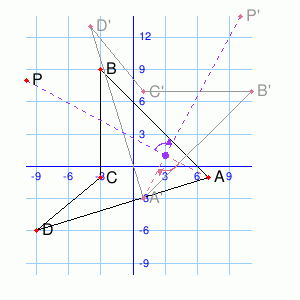Each point has been rotated around (3, 1) by -90°, which is also 270°.
A(7, -1) → A'(1, -3)
P(-10, 8) → P'(10, 14)
Since this is a rotation by an increment of 90°, we can figure out P' graphically by forming a right triangle with its hypotenuse connecting the pivot and point P. This should make it easy to draw the rotated P'. Your before and after triangles should both have legs of length 13 and 7, since P(-10, 8) is 13 from pivot (3, 1) in the x direction and 7 from it in the y direction.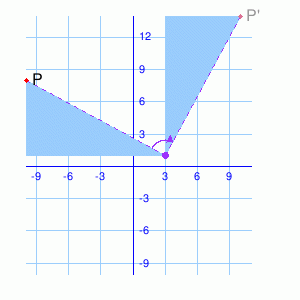### Complexity=2, Mode=refl

1Transformation ABCD → A'B'C'D' is a reflection across the line
e.g. y=-3, y=x.
Reflect P over the same line. (You may need to sketch things out on paper.)
P' = ( ,)
Solution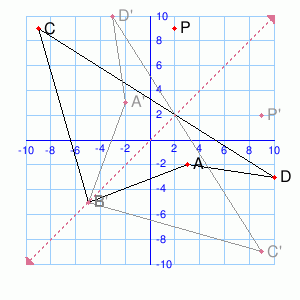P is at (2, 9). Since the line of reflection is y = x, reflecting a point comes down to switching the x and y coordinates.
2Transformation ABCD → A'B'C'D' is a reflection across the line
e.g. y=-3, y=x.
Reflect P over the same line. (You may need to sketch things out on paper.)
P' = ( ,)
Solution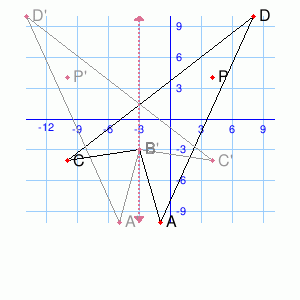The reflection is across x = -3.
P is at (4, 4). P' is at (_, 4).
Figure out the x-coordinate of P' by mirroring the distance (7) on the other side of the line.